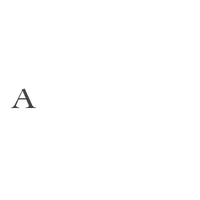Dressing up for your favourite occasions has never been easier. Sparkle across your day in our hand-picked range of jewels which are high on impact and low on pricing. From stackable rings to daily wear earrings, bracelets and dainty pendants, we have got you covered!

Rs. 120,000

Rs. 180,000

Rs. 130,000

Rs. 85,000

Rs. 140,000

Rs. 185,000

Rs. 90,000

Rs. 165,000

Rs. 142,000

Rs. 135,000

Rs. 115,000

Rs. 135,000

Rs. 175,000

Rs. 75,000

Rs. 40,000

Rs. 95,000

Rs. 85,000

Rs. 180,000

Rs. 140,000

Rs. 125,000

Rs. 135,000

Rs. 155,000

Rs. 168,000

Rs. 60,000

Rs. 120,000

Rs. 105,000

Rs. 110,000

Rs. 185,000

Rs. 185,000

Rs. 158,000

Rs. 192,000

Rs. 90,000

Rs. 188,000

Rs. 130,000

Rs. 120,000

Rs. 95,000

Rs. 120,000

Rs. 145,000

Rs. 110,000

Rs. 88,000

Rs. 75,000

Rs. 75,000

Rs. 105,000

Rs. 127,000

Rs. 95,000

Rs. 115,500

Rs. 75,000

Rs. 120,000

Rs. 120,000

Rs. 77,000

Rs. 93,000

Rs. 151,000

Rs. 115,500

Rs. 86,000

Rs. 115,000

Rs. 141,900

Rs. 66,000

Rs. 66,000

Rs. 138,000

Rs. 125,000

Rs. 96,000

Rs. 115,000

Rs. 65,000

Rs. 110,000

Rs. 95,000

Rs. 130,000

Rs. 70,000

Rs. 48,500

Rs. 36,000

Rs. 83,000

Rs. 155,000

Rs. 149,000

Rs. 130,000

Rs. 130,000

Rs. 133,000

Rs. 110,000

Rs. 75,000

Rs. 100,000

Rs. 155,000

Rs. 90,000

Rs. 150,000

Rs. 137,000